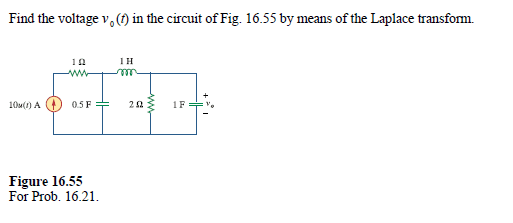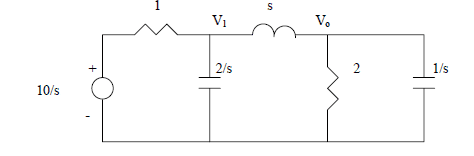# Nodal Analysis of this Circuit using the Laplace Transform

• Engineering
• jisbon
In summary, the answer booklet says that the current through the 1Ω resistor should be (10/s-V1)/1 = (V0-V1)/s + V1/(2/s), while the current through the 1 ohm resistor should be (10/s-V1)/1 = (V0-V1)/s + V0/(2/s).f

#### jisbon

Homework Statement
-
Relevant Equations
-
Was just practicing some problems on the Fundamentals of Electric Circuits, and came across this question.I understand I will have to transform to the s domain circuit, which looks something like this:Then doing nodal analysis, I will get the following for the first segement
(10/s-V1)/1 = (V0-V1)/s + V1/(2/s)

However, the answer booklet states that it should be: (10/s-V1)/1 = (V0-V1)/s + V0/(2/s)

I'm unsure if the answer booklet is wrong though,because I thought I was pretty confident in nodals until I see the answer.
What are the problems with my concepts in this case? Thanks

Last edited by a moderator:
Are you still treating it as a current source? It's hard to tell.

•cnh1995
I think you're both wrong.

Draw the current directions on your schematic at each node, make an equation at each node that sums the currents properly. Then substitute each current with the equation for the associated circuit component. Since you have 2 nodes, there will be 2 KCL equations (although from your question, it seems you only care about 1).

For example, the current through the 1 ohm resistor flowing from the source towards the inductor is (10/s-V1)/1, if you wanted the current in the other direction, it would be -(10/s-V1)/1 = (V1-10/s)/1. In both cases the current is the voltage difference across the element divided by the impedance of that element.

This is mostly an exercise in keeping careful track of the signs and adding things correctly.

BTW, The reason I know you answer book is wrong is the term Vo/(2/s). Vo isn't at that capacitor, there is no simple relationship between them.

@jisbon,
I believe you are considering the current source to be a voltage source in your calculations. It is evident from your node voltage equation (which would also be
incorrect if it were a voltage source).

Also, there is no switch in the circuit. It means you can assume the circuit to be already in steady state. I am not sure why Laplace transform is required here.

Oops, I missed the current source part. Yes, they are correct. The arrow means it's a current source.

So, then what is the current through the 1Ω resistor? Hint: Not what I said before, but your KCL equations in terms of the currents at each node are still the same, there's just a different substitution for the current through the 1Ω resistor.